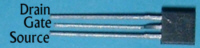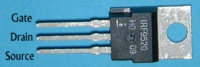#Field Effect transistors (FETs)

 Components Resistors Capacitors Inductors Crystals Diodes Transistors FETs Triacs Infra-red ICs LEDs Speakers and microphones Sensors

MOSFETs can be used to switch large currents. The voltage at the gate (VGS) of a MOSFET controls the amount of current that flow between the drain and the source (IDS). Almost no current flows into the gate of the MOSFET so a logic gate or op-amp can be connected to the gate and the MOSFET can switch on things that need a large current to work. The MOSFET is turned on when the p.d. between the gate and source is greater than the threshold voltage, VGS(TH)

When choosing a MOSFET you need to consider how much current needs to be switched and how much power is going to be dissipated in the MOSFET. The maximum current that can flow through a MOSFET is the saturation current, (IDSS). The Power in the MOSFET can be calculated from

P = VDS x IDS

When a FET is used for switching it is either on or off. When the MOSFET is off no power is dissipated because there is no current flowing. When the MOSFET is on it has a low resistance, RDS ON. This means there heat produced in the MOSFET which can bea calculated from
P = IDS2 x RDS ON

If a large amount of power needs to be dissipated then a heat sink should be used.

Small signal n-channel MOSFET BS170Philips Semiconductors
Large signal n-channel MOSFET IRF520AInternational Rectifier
Large signal p-channel MOSFET IRF9520International Rectifier
Small signal n-channel Junction-FET BF244Fairchild Semiconductor